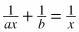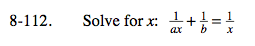Home > A2C > Chapter 8 > Lesson 8.1.7 > Problem8-112

8-112.

Solve for x:. Homework Help ✎$\text{First multiply the equation by }\frac{abx}{abx}.$

b + ax = ab

$x=\frac{ab-b}{a}$